## Section 4.5: Graphs of the Sine and Cosine Function

### Learning Outcomes

• Determine amplitude, period, phase shift, and vertical shift of a sine or cosine graph from its equation.
• Graph variations of y=cos x and y=sin x .
• Determine a function formula that would have a given sinusoidal graph.
• Determine functions that model circular and periodic motion.

## Graph variations of  y=sin( x )  and  y=cos( x )

Recall that the sine and cosine functions relate real number values to the x– and y-coordinates of a point on the unit circle. So what do they look like on a graph on a coordinate plane? Let’s start with the sine function. We can create a table of values and use them to sketch a graph. The table below lists some of the values for the sine function on a unit circle.

 x 0 $\frac{\pi}{6}$ $\frac{\pi}{4}$ $\frac{\pi}{3}$ $\frac{\pi}{2}$ $\frac{2\pi}{3}$ $\frac{3\pi}{4}$ $\frac{5\pi}{6}$ $\pi$ $\sin(x)$ 0 $\frac{1}{2}$ $\frac{\sqrt{2}}{2}$ $\frac{\sqrt{3}}{2}$ 1 $\frac{\sqrt{3}}{2}$ $\frac{\sqrt{2}}{2}$ $\frac{1}{2}$ 0

Plotting the points from the table and continuing along the x-axis gives the shape of the sine function. See Figure 2.

Notice how the sine values are positive between 0 and π, which correspond to the values of the sine function in quadrants I and II on the unit circle, and the sine values are negative between π and 2π, which correspond to the values of the sine function in quadrants III and IV on the unit circle. See Figure 3.

Now let’s take a similar look at the cosine function. Again, we can create a table of values and use them to sketch a graph. The table below lists some of the values for the cosine function on a unit circle.

 x 0 $\frac{\pi}{6}$ $\frac{\pi}{4}$ $\frac{\pi}{3}$ $\frac{\pi}{2}$ $\frac{2\pi}{3}$ $\frac{3\pi}{4}$ $\frac{5\pi}{6}$ $\pi$ $\cos(x)$ 1 $\frac{\sqrt{3}}{2}$ $\frac{\sqrt{2}}{2}$ $\frac{1}{2}$ 0 $-\frac{1}{2}$ $-\frac{\sqrt{2}}{2}$ $-\frac{\sqrt{3}}{2}$ −1

As with the sine function, we can plots points to create a graph of the cosine function as in Figure 4.

Because we can evaluate the sine and cosine of any real number, both of these functions are defined for all real numbers. By thinking of the sine and cosine values as coordinates of points on a unit circle, it becomes clear that the range of both functions must be the interval [−1,1].

In both graphs, the shape of the graph repeats after 2π,which means the functions are periodic with a period of $2π$. A periodic function is a function for which a specific horizontal shift, P, results in a function equal to the original function: $f (x + P) = f(x)$ for all values of x in the domain of f. When this occurs, we call the smallest such horizontal shift with $P > 0$ the period of the function. Figure 5 shows several periods of the sine and cosine functions.

Looking again at the sine and cosine functions on a domain centered at the y-axis helps reveal symmetries. As we can see in Figure 6, the sine function is symmetric about the origin. Recall from Section 6.2: Trigonometric Functions: Unit Circle Approach that we determined from the unit circle that the sine function is an odd function because $\sin(−x)=−\sin x$. Now we can clearly see this property from the graph.

Figure 7 shows that the cosine function is symmetric about the y-axis. Again, we determined that the cosine function is an even function. Now we can see from the graph that $\cos(−x)=\cos x$.

### A General Note: Characteristics of Sine and Cosine Functions

The sine and cosine functions have several distinct characteristics:

• They are periodic functions with a period of 2π.
• The domain of each function is $\left(-\infty,\infty\right)$ and the range is $\left[−1,1\right]$.
• The graph of $y=\sin x$ is symmetric about the origin, because it is an odd function.
• The graph of $y=\cos x$ is symmetric about the y-axis, because it is an even function.

## Investigating Sinusoidal Functions

As we can see, sine and cosine functions have a regular period and range. If we watch ocean waves or ripples on a pond, we will see that they resemble the sine or cosine functions. However, they are not necessarily identical. Some are taller or longer than others. A function that has the same general shape as a sine or cosine function is known as a sinusoidal function. The general forms of sinusoidal functions are

$y = A\sin (Bx−C) + D$

and

$y = A\cos (Bx−C) + D$

### Determining the Period of Sinusoidal Functions

Looking at the forms of sinusoidal functions, we can see that they are transformations of the sine and cosine functions. We can use what we know about transformations to determine the period.

In the general formula, B is related to the period by $P=\frac{2π}{|B|}$. If $|B| > 1$, then the period is less than $2π$ and the function undergoes a horizontal compression, whereas if $|B| < 1$, then the period is greater than $2π$ and the function undergoes a horizontal stretch. For example, $f(x) = \sin(x), B= 1$, so the period is $2π$, which we knew. If $f(x) =\sin (2x)$, then $B= 2$, so the period is $π$ and the graph is compressed. If $f(x) = \sin\left(\frac{x}{2} \right)$, then $B=\frac{1}{2}$, so the period is $4π$ and the graph is stretched. Notice in Figure 8 how the period is indirectly related to $|B|$.

### A General Note: Period of Sinusoidal Functions

If we let C = 0 and D = 0 in the general form equations of the sine and cosine functions, we obtain the forms

$y=A\sin\left(Bx\right)$

$y=A\cos\left(Bx\right)$

The period is $\frac{2π}{|B|}$.

### Example 1: Identifying the Period of a Sine or Cosine Function

Determine the period of the function $f(x) = \sin\left(\frac{π}{6}x\right)$.

### Try It

Determine the period of the function $g(x)=\cos\left(\frac{x}{3}\right)$.

### Determining Amplitude

Returning to the general formula for a sinusoidal function, we have analyzed how the variable B relates to the period. Now let’s turn to the variable A so we can analyze how it is related to the amplitude, or greatest distance from rest. A represents the vertical stretch factor, and its absolute value |A| is the amplitude. The local maxima will be a distance |A| above the vertical midline of the graph, which is the line = D; because D = 0 in this case, the midline is the x-axis. The local minima will be the same distance below the midline. If |A| > 1, the function is stretched. For example, the amplitude of $f(x)=4\sin\left(x\right)$ is twice the amplitude of

$f(x)=2\sin\left(x\right)$

If $|A| < 1$, the function is compressed. Figure 9 compares several sine functions with different amplitudes.

### A General Note: Amplitude of Sinusoidal Functions

If we let = 0 and D = 0 in the general form equations of the sine and cosine functions, we obtain the forms

$y=A\sin(Bx)$ and $y=A\cos(Bx)$

The amplitude is A, and the vertical height from the midline is |A|. In addition, notice in the example that

$|A|=\text{amplitude}=\frac{1}{2}|\text{maximum}−\text{minimum}|$

### Example 2: Identifying the Amplitude of a Sine or Cosine Function

What is the amplitude of the sinusoidal function $f(x)=−4\sin(x)$? Is the function stretched or compressed vertically?

### Try It

What is the amplitude of the sinusoidal function $f(x)=12\sin (x)$? Is the function stretched or compressed vertically?

## Analyzing Graphs of Variations of y = sin x and y = cos x

Now that we understand how A and B relate to the general form equation for the sine and cosine functions, we will explore the variables and D. Recall the general form:

$y = A \sin(Bx−C)+D$ and $y=A\cos(Bx−C)+D$
or
$y=A\sin(B(x−\frac{C}{B}))+D$ and $y=A\cos(B(x−\frac{C}{B}))+D$

The value $\frac{C}{B}$ for a sinusoidal function is called the phase shift, or the horizontal displacement of the basic sine or cosine function. If C > 0, the graph shifts to the right. If C < 0,the graph shifts to the left. The greater the value of |C|, the more the graph is shifted. Figure 11 shows that the graph of $f(x)=\sin(x−π)$ shifts to the right by π units, which is more than we see in the graph of $f(x)=\sin(x−\frac{π}{4})$, which shifts to the right by $\frac{π}{4}$units.

While C relates to the horizontal shift, D indicates the vertical shift from the midline in the general formula for a sinusoidal function. The function $y=\cos(x)+D$ has its midline at $y=D$.

Any value of D other than zero shifts the graph up or down. Figure 13 compares $f(x)=\sin x$ with $f(x)=\sin (x)+2$, which is shifted 2 units up on a graph.

### A General Note: Variations of Sine and Cosine Functions

Given an equation in the form $f(x)=A\sin(Bx−C)+D$ or $f(x)=A\cos(Bx−C)+D$, $\frac{C}{B}$is the phase shift and D is the vertical shift.

### Example 3: Identifying the Phase Shift of a Function

Determine the direction and magnitude of the phase shift for $f(x)=\sin(x+\frac{π}{6})−2$.

### Try It

Determine the direction and magnitude of the phase shift for $f(x)=3\cos(x−\frac{\pi}{2})$.

### Example 4: Identifying the Vertical Shift of a Function

Determine the direction and magnitude of the vertical shift for $f(x)=\cos(x)−3$.

### Try It

Determine the direction and magnitude of the vertical shift for $f(x)=3\sin(x)+2$.

### How To: Given a sinusoidal function in the form $f(x)=A\sin(Bx−C)+D$, identify the midline, amplitude, period, and phase shift.

1. Determine the amplitude as |A|.
2. Determine the period as $P=\frac{2π}{|B|}$.
3. Determine the phase shift as $\frac{C}{B}$.
4. Determine the midline as = D.

### Example 5: Identifying the Variations of a Sinusoidal Function from an Equation

Determine the midline, amplitude, period, and phase shift of the function $y=3\sin(2x)+1$.

### Try It

Determine the midline, amplitude, period, and phase shift of the function $y=\frac{1}{2}\cos(\frac{x}{3}−\frac{π}{3})$.

### Example 6: Identifying the Equation for a Sinusoidal Function from a Graph

Determine the formula for the cosine function in Figure 15.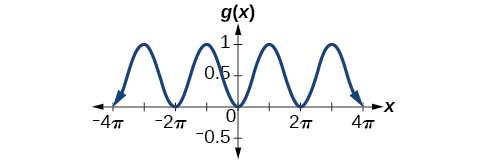Figure 15

### Try It

Determine the formula for the sine function in Figure 16.

### Example 7: Identifying the Equation for a Sinusoidal Function from a Graph

Determine the equation for the sinusoidal function in Figure 17.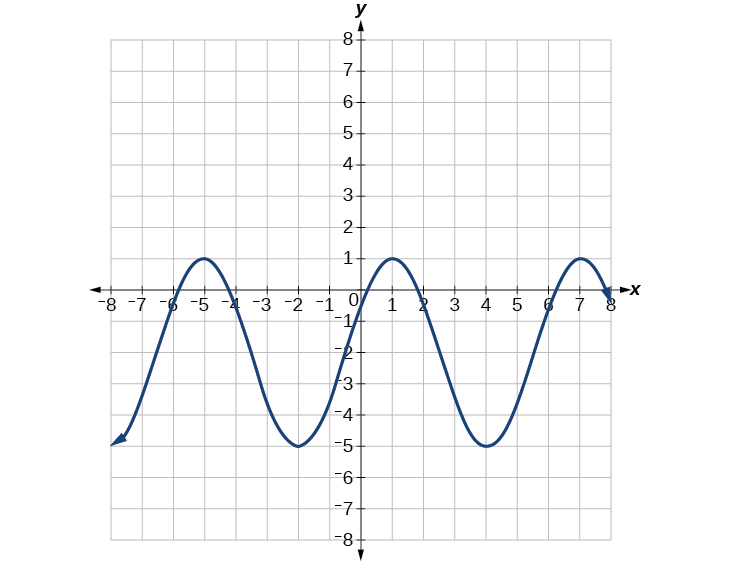Figure 17

### Try It

Write a formula for the function graphed in Figure 18.

## Graphing Variations of y = sin x and y = cos x

Throughout this section, we have learned about types of variations of sine and cosine functions and used that information to write equations from graphs. Now we can use the same information to create graphs from equations.

Instead of focusing on the general form equations

$y=A\sin(Bx−C)+D$ and $y=A\cos(Bx−C)+D$,

we will let C = 0 and D = 0 and work with a simplified form of the equations in the following examples.

### How To: Given the function $y=Asin(Bx)$, sketch its graph.

1. Identify the amplitude,|A|.
2. Identify the period, $P=\frac{2π}{|B|}$.
3. Start at the origin, with the function increasing to the right if A is positive or decreasing if A is negative.
4. At $x=\frac{π}{2|B|}$ there is a local maximum for A > 0 or a minimum for A < 0, with y = A.
5. The curve returns to the x-axis at $x=\frac{π}{|B|}$.
6. There is a local minimum for A > 0 (maximum for < 0) at $x=\frac{3π}{2|B|}$ with = –A.
7. The curve returns again to the x-axis at $x=\frac{π}{2|B|}$.

### Example 8: Graphing a Function and Identifying the Amplitude and Period

Sketch a graph of $f(x)=−2\sin(\frac{πx}{2})$.

### Try It

Sketch a graph of $g(x)=−0.8\cos(2x)$. Determine the midline, amplitude, period, and phase shift.

### How To: Given a sinusoidal function with a phase shift and a vertical shift, sketch its graph.

1. Express the function in the general form $y=A\sin(Bx−C)+D$ or $y=A\cos(Bx−C)+D$.
2. Identify the amplitude, |A|.
3. Identify the period, $P=2π|B|$.
4. Identify the phase shift, $\frac{C}{B}$.
5. Draw the graph of $f(x)=A\sin(Bx)$ shifted to the right or left by $\frac{C}{B}$ and up or down by D.

### Example 9: Graphing a Transformed Sinusoid

Sketch a graph of $f(x)=3\sin\left(\frac{π}{4}x−\frac{π}{4}\right)$.

### Try It

Draw a graph of $g(x)=−2\cos(\frac{\pi}{3}x+\frac{\pi}{6})$. Determine the midline, amplitude, period, and phase shift.

### Example 10: Identifying the Properties of a Sinusoidal Function

Given $y=−2\cos\left(\frac{\pi}{2}x+\pi\right)+3$, determine the amplitude, period, phase shift, and horizontal shift. Then graph the function.

## Using Transformations of Sine and Cosine Functions

We can use the transformations of sine and cosine functions in numerous applications. As mentioned at the beginning of the chapter, circular motion can be modeled using either the sine or cosine function.

### Example 11: Finding the Vertical Component of Circular Motion

A point rotates around a circle of radius 3 centered at the origin. Sketch a graph of the y-coordinate of the point as a function of the angle of rotation.

### Try It

What is the amplitude of the function $f(x)=7\cos(x)$? Sketch a graph of this function.

### Example 12: Finding the Vertical Component of Circular Motion

A circle with radius 3 ft is mounted with its center 4 ft off the ground. The point closest to the ground is labeled P, as shown in Figure 23. Sketch a graph of the height above the ground of the point P as the circle is rotated; then find a function that gives the height in terms of the angle of rotation.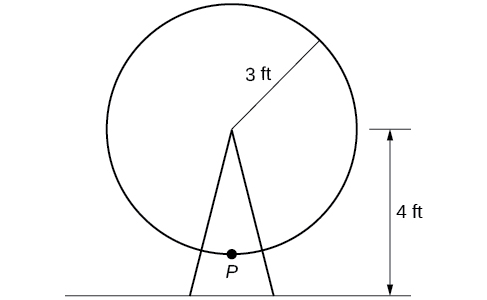Figure 23

### Try It

A weight is attached to a spring that is then hung from a board, as shown in Figure 25. As the spring oscillates up and down, the position y of the weight relative to the board ranges from –1 in. (at time x = 0) to –7in. (at time x = π) below the board. Assume the position of y is given as a sinusoidal function of x. Sketch a graph of the function, and then find a cosine function that gives the position y in terms of x.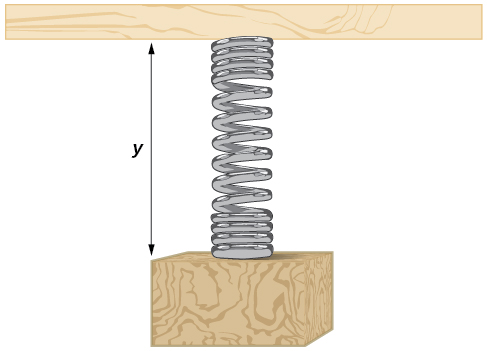Figure 25

### Example 13: Determining a Rider’s Height on a Ferris Wheel

The London Eye is a huge Ferris wheel with a diameter of 135 meters (443 feet). It completes one rotation every 30 minutes. Riders board from a platform 2 meters above the ground. Express a rider’s height above ground as a function of time in minutes.

### Try It

Key Equations

 Sinusoidal functions $f(x)=A\sin(Bx−C)+D$ $f(x)=A\cos(Bx−C)+D$

# Key Concepts

• Periodic functions repeat after a given value. The smallest such value is the period. The basic sine and cosine functions have a period of 2π.
• The function sin x is odd, so its graph is symmetric about the origin. The function cos x is even, so its graph is symmetric about the y-axis.
• The graph of a sinusoidal function has the same general shape as a sine or cosine function.
• In the general formula for a sinusoidal function, the period is $\text{P}=\frac{2\pi}{|B|}$.
• In the general formula for a sinusoidal function, |A|represents amplitude. If |A| > 1, the function is stretched, whereas if|A| < 1, the function is compressed.
• The value $\frac{C}{B}$ in the general formula for a sinusoidal function indicates the phase shift.
• The value D in the general formula for a sinusoidal function indicates the vertical shift from the midline.
• Combinations of variations of sinusoidal functions can be detected from an equation.
• The equation for a sinusoidal function can be determined from a graph.
• A function can be graphed by identifying its amplitude and period.
• A function can also be graphed by identifying its amplitude, period, phase shift, and horizontal shift.
• Sinusoidal functions can be used to solve real-world problems.

## Glossary

amplitude
the vertical height of a function; the constant A appearing in the definition of a sinusoidal function
midline
the horizontal line = D, where D appears in the general form of a sinusoidal function
periodic function
a function f(x) that satisfies $f(x+P)=f(x)$ for a specific constant and any value of x
phase shift
the horizontal displacement of the basic sine or cosine function; the constant $\frac{C}{B}$
sinusoidal function
any function that can be expressed in the form $f(x)=A\sin(Bx−C)+D$ or $f(x)=A\cos(Bx−C)+D$

## Section 4.5 Homework Exercises

1. Why are the sine and cosine functions called periodic functions?

2. How does the graph of $y=\sin x$ compare with the graph of $y=\cos x$? Explain how you could horizontally translate the graph of $y=\sin x$ to obtain $y=\cos x$.

3. For the equation $A\cos(Bx+C)+D$, what constants affect the range of the function and how do they affect the range?

4. How does the range of a translated sine function relate to the equation $y=A\sin(Bx+C)+D$?

5. How can the unit circle be used to construct the graph of $f(t)=\sin t$?

For the following exercises, graph each function. Identify the amplitude, period, midline, maximum, minimum, and key points.

6. $f(x)=2\sin x$

7. $f(x)=\frac{2}{3}\cos x$

8. $f(x)=−3\sin x$

9. $f(x)=4\sin x$

10. $f(x)=2\cos x$

11. $f(x)=\cos(2x)$

12. $f(x)=2\sin\left(\frac{1}{2}x\right)$

13. $f(x)=4\cos(\pi x)$

14. $f(x)=3\cos\left(\frac{6}{5}x\right)$

15. $y=3\sin(8(x+4))+5$

16. $y=2\sin(3x−21)+4$

17. $y=5\sin(5x+20)−2$

For the following exercises, graph one full period of each function, starting at $x=0$. For each function, state the amplitude, period, and midline. State the maximum and minimum y-values and their corresponding x-values on one period for $x>0$. State the phase shift and vertical translation, if applicable. Round answers to two decimal places if necessary.

18. $f(t)=2\sin\left(t−\frac{5\pi}{6}\right)$

19. $f(t)=−\cos\left(t+\frac{\pi}{3}\right)+1$

20. $f(t)=4\cos\left(2\left(t+\frac{\pi}{4}\right)\right)−3$

21. $f(t)=−\sin\left(12t+\frac{5\pi}{3}\right)$

22. $f(x)=4\sin\left(\frac{\pi}{2}(x−3)\right)+7$

23. Determine the amplitude, midline, period, and an equation involving the sine function for the graph shown in Figure 26.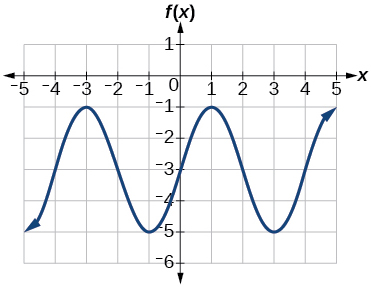Figure 26

24. Determine the amplitude, period, midline, and an equation involving cosine for the graph shown in Figure 27.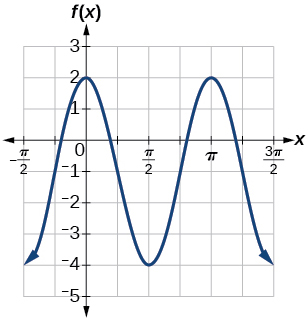Figure 27

25. Determine the amplitude, period, midline, and an equation involving cosine for the graph shown in Figure 28.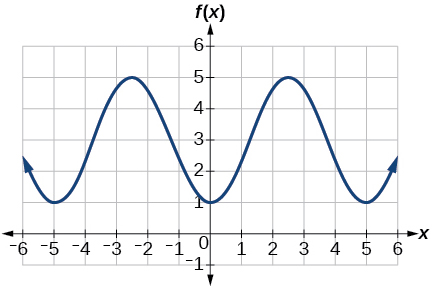Figure 28

26. Determine the amplitude, period, midline, and an equation involving sine for the graph shown in Figure 29.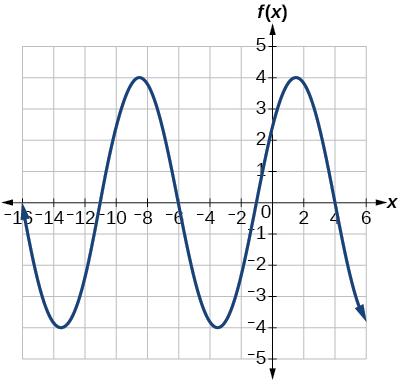Figure 29

27. Determine the amplitude, period, midline, and an equation involving cosine for the graph shown in Figure 30.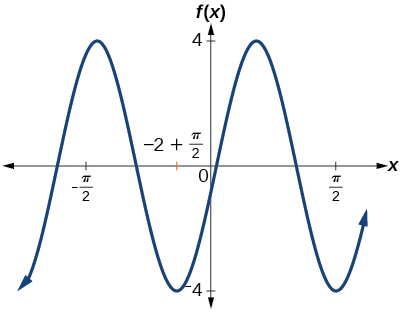Figure 30

28. Determine the amplitude, period, midline, and an equation involving sine for the graph shown in Figure 31.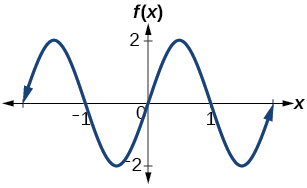Figure 31

29. Determine the amplitude, period, midline, and an equation involving cosine for the graph shown in Figure 32.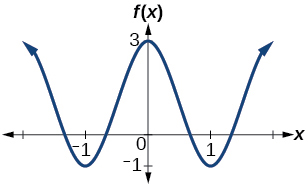Figure 32

30. Determine the amplitude, period, midline, and an equation involving sine for the graph shown in Figure 33.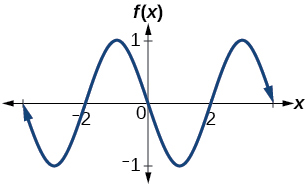Figure 33

For the following exercises, let $f(x)=\sin x$.

31. On [0,2π), solve $f(x)=\frac{1}{2}$.

32. Evaluate $f\left(\frac{\pi}{2}\right)$.

33. On [0,2π), $f(x)=\frac{\sqrt{2}}{2}$. Find all values of x.

34. On [0,2π), the maximum value(s) of the function occur(s) at what x-value(s)?

35. On [0,2π), the minimum value(s) of the function occur(s) at what x-value(s)?

36. Show that $f(−x)=−f(x)$.This means that $f(x)=\sin x$ is an odd function and possesses symmetry with respect to ________________.

For the following exercises, let $f(x)=\cos x$.

37. On [0,2π), solve the equation $f(x)=\cos x=0$.

38. On[0,2π), solve $f(x)=\frac{1}{2}$.

39. On [0,2π), find the x-intercepts of $f(x)=\cos x$.

40. On [0,2π), find the x-values at which the function has a maximum or minimum value.

41. On [0,2π), solve the equation $f(x)=\frac{\sqrt{3}}{2}$.

42. Graph $h(x)=x+\sin x \text{ on}[0,2\pi]$. Explain why the graph appears as it does.

43. Graph $h(x)=x+\sin x$ on[−100,100]. Did the graph appear as predicted in the previous exercise?

44. Graph $f(x)=x\sin x$ on [0,2π] and verbalize how the graph varies from the graph of $f(x)=\sin x$.

45. Graph $f(x)=x\sin x$ on the window [−10,10] and explain what the graph shows.

46. Graph $f(x)=\frac{\sin x}{x}$ on the window [−5π,5π] and explain what the graph shows.

47. A Ferris wheel is 25 meters in diameter and boarded from a platform that is 1 meter above the ground. The six o’clock position on the Ferris wheel is level with the loading platform. The wheel completes 1 full revolution in 10 minutes. The function h(t) gives a person’s height in meters above the ground t minutes after the wheel begins to turn.
a. Find the amplitude, midline, and period of h(t).
b. Find a formula for the height function h(t).
c. How high off the ground is a person after 5 minutes?